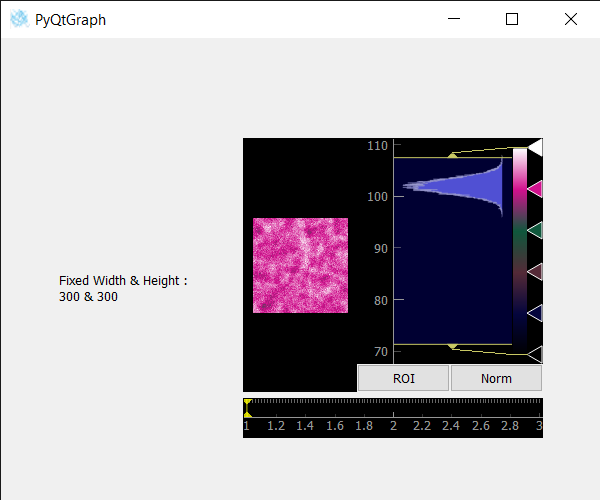# PyQtGraph – Getting Fixed Width/Height of Image View

• Last Updated : 26 May, 2022

In this article, we will see how we can get the fixed width/height of the image view in PyQTGraph. PyQtGraph is a graphics and user interface library for Python that provides functionality commonly required in designing and science applications. Its primary goals are to provide fast, interactive graphics for displaying data (plots, video, etc.). Widget used for display and analysis of image data. Implements many features like displaying 2D and 3D image data. For 3D data, a z-axis slider is displayed allowing the user to select which frame is displayed. Displays histogram of image data with movable region defining the dark/light levels, editable gradient provides a color lookup table. Fixed width/height is the fixed length which can not be decreased or increased by default it is not set to the image view, it can be set with the help of setFixedWidth() and setFixedHeight() method.
We can create an image view with the help of the command given below

```# creating a pyqtgraph image view object
imv = pg.ImageView()```

Syntax: ImageView(parent=None, name=’ImageView’, view=None, imageItem=None, levelMode=’mono’, *args)

Parameters:

• parent (QWidget): Specifies the parent widget to which this ImageView will belong. If None, then the ImageView is created with no parent.
• name (str): The name used to register both the internal ViewBox and the PlotItem used to display ROI data.
• view (ViewBox or PlotItem): If specified, this will be used as the display area that contains the displayed image.
• imageItem (ImageItem): If specified, this object will be used to display the image. Must be an instance of ImageItem or other compatible object.
• levelMode: specifies the *levelMode* argument

Returns: Object of class ImageView

In order to do get the width and height we use width() and height() method respectively with the image view object

Syntax : imv.width() and imv.height()
Argument : It takes no argument
Return : It returns integer

Below is the implementation

## Python3

 `# importing Qt widgets` `from` `PyQt5.QtWidgets ``import` `*`   `# importing system` `import` `sys`   `# importing numpy as np` `import` `numpy as np`   `# importing pyqtgraph as pg` `import` `pyqtgraph as pg` `from` `PyQt5.QtGui ``import` `*` `from` `PyQt5.QtCore ``import` `*`   `# Image View class` `class` `ImageView(pg.ImageView):`   `    ``# constructor which inherit original` `    ``# ImageView` `    ``def` `__init__(``self``, ``*``args, ``*``*``kwargs):` `        ``pg.ImageView.__init__(``self``, ``*``args, ``*``*``kwargs)`   `class` `Window(QMainWindow):`   `    ``def` `__init__(``self``):` `        ``super``().__init__()`   `        ``# setting title` `        ``self``.setWindowTitle(``"PyQtGraph"``)`   `        ``# setting geometry` `        ``self``.setGeometry(``100``, ``100``, ``600``, ``500``)`   `        ``# icon` `        ``icon ``=` `QIcon(``"skin.png"``)`   `        ``# setting icon to the window` `        ``self``.setWindowIcon(icon)`   `        ``# calling method` `        ``self``.UiComponents()`   `        ``# showing all the widgets` `        ``self``.show()`   `    ``# method for components` `    ``def` `UiComponents(``self``):`   `        ``# creating a widget object` `        ``widget ``=` `QWidget()`   `        ``# creating a label` `        ``label ``=` `QLabel(``"Geeksforgeeks Image View"``)`   `        ``# setting minimum width` `        ``label.setMinimumWidth(``130``)`   `        ``# making label do word wrap` `        ``label.setWordWrap(``True``)`   `        ``# setting configuration options` `        ``pg.setConfigOptions(antialias ``=` `True``)`   `        ``# creating image view  object` `        ``imv ``=` `ImageView()`   `        ``# Create random 3D data set with noisy signals` `        ``img ``=` `pg.gaussianFilter(np.random.normal(size``=``(``200``, ``200``)), ` `                                ``(``5``, ``5``)) ``*` `20` `+` `100`   `        ``# setting new axis to image` `        ``img ``=` `img[np.newaxis, :, :]`   `        ``# decay data` `        ``decay ``=` `np.exp(``-``np.linspace(``0``, ``0.3``, ``100``))[:, np.newaxis, np.newaxis]`   `        ``# random data` `        ``data ``=` `np.random.normal(size``=``(``100``, ``200``, ``200``))` `        ``data ``+``=` `img ``*` `decay` `        ``data ``+``=` `2`   `        ``# adding time-varying signal` `        ``sig ``=` `np.zeros(data.shape[``0``])` `        ``sig[``30``:] ``+``=` `np.exp(``-``np.linspace(``1``, ``10``, ``70``))` `        ``sig[``40``:] ``+``=` `np.exp(``-``np.linspace(``1``, ``10``, ``60``))` `        ``sig[``70``:] ``+``=` `np.exp(``-``np.linspace(``1``, ``10``, ``30``))`   `        ``sig ``=` `sig[:, np.newaxis, np.newaxis] ``*` `3` `        ``data[:, ``50``:``60``, ``30``:``40``] ``+``=` `sig`   `        ``# setting image to image view` `        ``# Displaying the data and assign each frame a time value from 1.0 to 3.0` `        ``imv.setImage(data, xvals``=``np.linspace(``1.``, ``3.``, data.shape[``0``]))`   `        ``# Set a custom color map` `        ``colors ``=` `[` `            ``(``0``, ``0``, ``0``),` `            ``(``4``, ``5``, ``61``),` `            ``(``84``, ``42``, ``55``),` `            ``(``15``, ``87``, ``60``),` `            ``(``208``, ``17``, ``141``),` `            ``(``255``, ``255``, ``255``)` `        ``]`   `        ``# color map` `        ``cmap ``=` `pg.ColorMap(pos``=``np.linspace(``0.0``, ``1.0``, ``6``), color ``=` `colors)`   `        ``# setting color map to the image view` `        ``imv.setColorMap(cmap)`   `        ``# Creating a grid layout` `        ``layout ``=` `QGridLayout()`   `        ``# minimum width value of the label` `        ``label.setFixedWidth(``130``)`   `        ``# setting this layout to the widget` `        ``widget.setLayout(layout)`   `        ``# adding label in the layout` `        ``layout.addWidget(label, ``1``, ``0``)`   `        ``# plot window goes on right side, spanning 3 rows` `        ``layout.addWidget(imv, ``0``, ``1``, ``3``, ``1``)`   `        ``# setting this widget as central widget of the main window` `        ``self``.setCentralWidget(widget)`   `        ``# setting fixed width and height` `        ``imv.setFixedWidth(``300``)` `        ``imv.setFixedHeight(``300``)`   `        ``# getting fixed width and height of image view` `        ``value1 ``=` `imv.width()` `        ``value2 ``=` `imv.height()`   `        ``# setting text to the label` `        ``label.setText(``"Fixed Width & Height : "` `+` `str``(value1) ``+` `" & "` `+` `str``(value2))`   `# create pyqt5 app` `App ``=` `QApplication(sys.argv)`   `# create the instance of our Window` `window ``=` `Window()`   `# start the app` `sys.exit(App.``exec``())`

Output :My Personal Notes arrow_drop_up
Recommended Articles
Page :